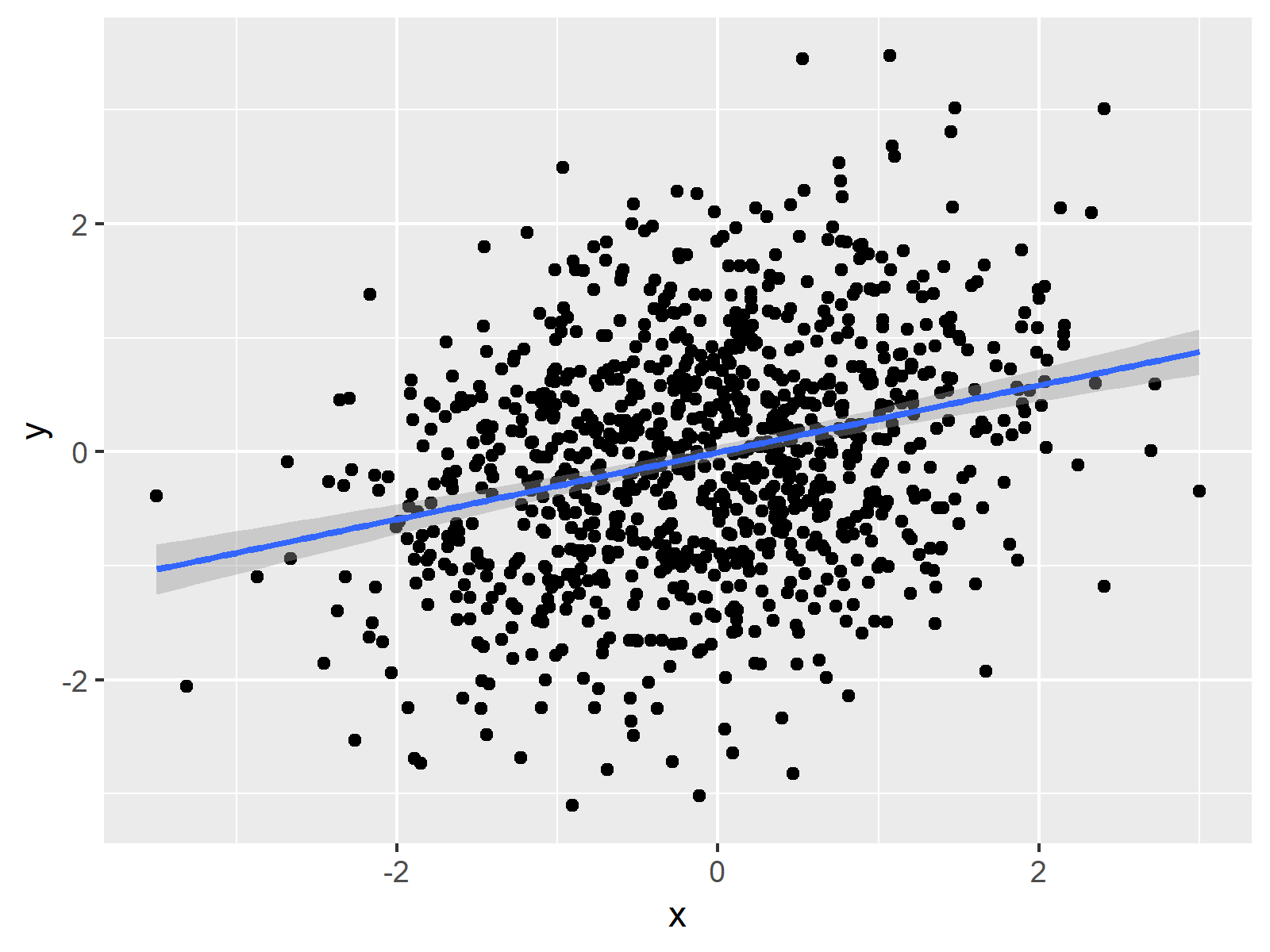# Add Regression Line to ggplot2 Plot in R (Example) | Draw Linear Slope to Scatterplot

In this R tutorial you’ll learn how to add regression lines on scatterplots.

The article contains one examples for the addition of a regression slope. More precisely, the content of the tutorial looks as follows:

With that, let’s start right away.

## Creation of Example Data

In the following R programming tutorial, we’ll use the data frame below as basement:

```set.seed(8743)                             # Create example data
x <- rnorm(1000)
y <- rnorm(1000) + 0.3 * x
data <- data.frame(x, y)
head(data)                                 # Print first rows of data
#            x          y
# 1  1.2138865 -0.3500503
# 2 -1.8828867 -1.1576045
# 3 -0.2739309 -0.9035707
# 4  1.0028479 -0.1521459
# 5  0.6276009 -0.4914815
# 6 -0.9443908 -1.3845497```

As you can see based on the output of the RStudio console, our example data contains two numeric columns x and y.

Furthermore, we have to install and load the ggplot2 package to R:

```install.packages("ggplot2")                # Install & load ggplot2
library("ggplot2")```

Now, we can draw a basic scatterplot with the ggplot2 package with the ggplot & geom_point functions as follows:

```ggp <- ggplot(data, aes(x, y)) +           # Create basic ggplot
geom_point()
ggp                                        # Print ggplot```Figure 1: Basic ggplot2 Scatterplot without Regression Line.

Figure 1 shows the graphic that we have just created. It’s a simple dotplot showing the correlation of our variables x and y.

## Example 1: Adding Linear Regression Line to Scatterplot

As you have seen in Figure 1, our data is correlated. We may want to draw a regression slope on top of our graph to illustrate this correlation.

With the ggplot2 package, we can add a linear regression line with the geom_smooth function. Have a look at the following R code:

```ggp +                                      # Add regression line
geom_smooth(method = "lm",
formula = y ~ x)```Figure 2: ggplot2 Scatterplot with Linear Regression Line and Variance.

Figure 2 shows our updated plot. As you can see, it consists of the same data points as Figure 1 and in addition it shows the linear regression slope corresponding to our data values. The shaded area around the trend line illustrates the variance.

## Video, Further Resources & Summary

Do you want to know more about regression slopes and graphics in R? Then you may watch the following video which I have published on my YouTube channel. In the video, I’m explaining the R programming codes of this tutorial.

Please accept YouTube cookies to play this video. By accepting you will be accessing content from YouTube, a service provided by an external third party.If you accept this notice, your choice will be saved and the page will refresh.

Besides the video, you may want to read the other tutorials of my website. You can find some tutorials here.

In summary: In this post, I showed how to insert a linear regression equation line to a ggplot2 graph in R. In case you have any additional questions, let me know in the comments section.

Subscribe to the Statistics Globe Newsletter

•Thomas.Kroeber
September 27, 2022 7:47 am

How can I add co-factors to a linear model “lm” when I insert a linear regression equation line to a ggplot2 graph ?

•September 30, 2022 5:41 pm